Latest Banking jobs   »   IBPS RRB quant

# Quantitative Aptitude Quiz For IBPS RRB Clerk Mains 2023-11th-September

Directions (1-5): In each question two equations numbered (I) and (II) are given. Student should solve both the equations and mark appropriate answer.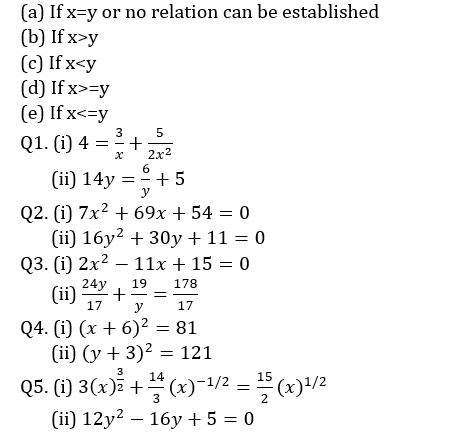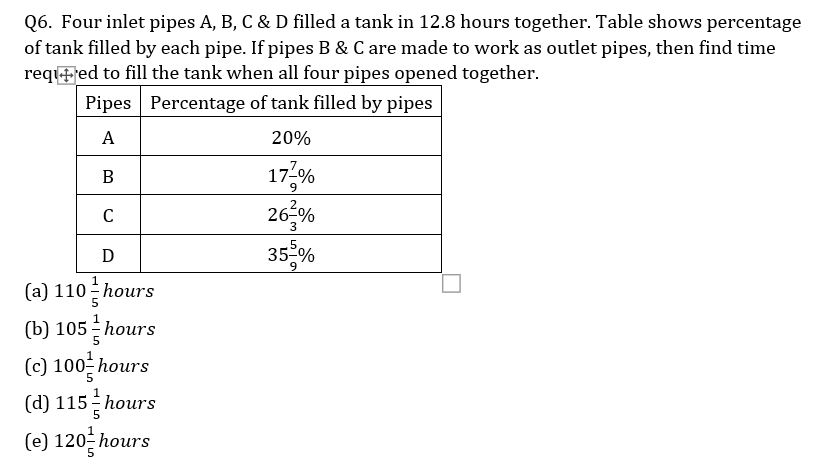Q7.The ratio of average of two series of five numbers each is 2:3. All five terms of the former series are consecutive multiples of 4 while all five terms of the latter series are consecutive multiples of 6. If sum of their first term is 20, then find the difference between greatest term of series divisible by 6 and second greatest term of series divisible by 4?
(a) 16
(b) 12
(c) 10
(d) 14
(e) 8

Q8.A sum of Rs ‘x’ becomes Rs (x + 528) in two year at the rate of 20% p.a compounded annually. if Veer invested Rs 2.5x at 15% p.a. on SI for three years and Ayush invest Rs 1.5x at 10% on CI for two years, then find the ratio of interest got by Ayush to that of Veer?
(a) 4 : 25
(b) 6 : 25
(c) 8 : 25
(d) 7 : 25
(e) None of these

Q9. Average of present ages of A, B and C is 35 years. 3 years hence, C is twice as old as A, while 3 years ago sum of ages of B and C is twice of present age of B. Then, find the ratio of present age of A, B and C.
(a) 7 : 13 : 15
(b) 11 : 14 : 16
(c) 3 : 6 : 8
(d) 9 : 13 : 15
(e) 2 : 3 : 6

Q10. Deepak bought two different articles- A and B, total cost price of both articles is Rs. 6500. He had incurred an overall loss of Rs.25 by selling article A at a profit of 25% and article B at a loss of 30%. Then, find the cost price of article A?
(a) Rs.3500
(b) Rs.4300
(c) Rs.3600
(d) Rs.3100
(e) None of the above.

Solutions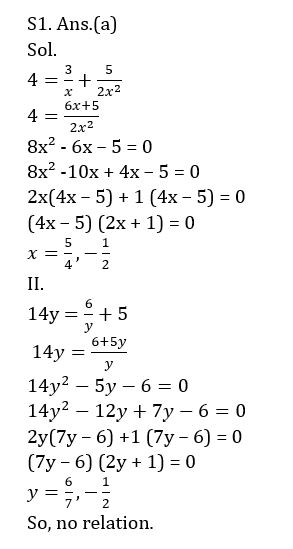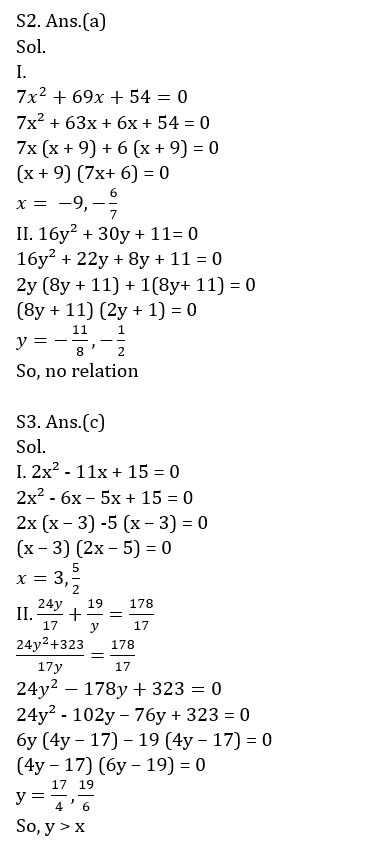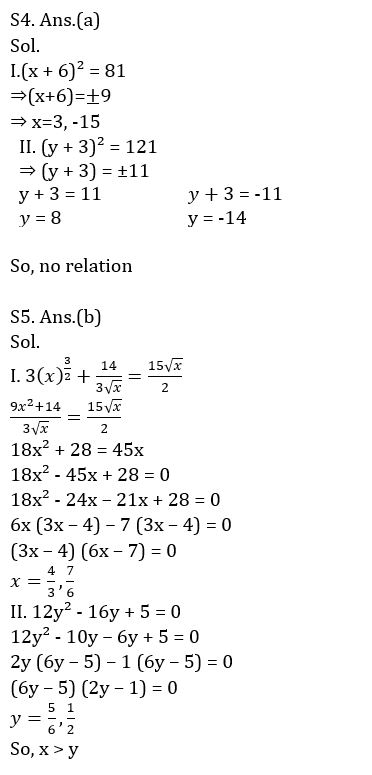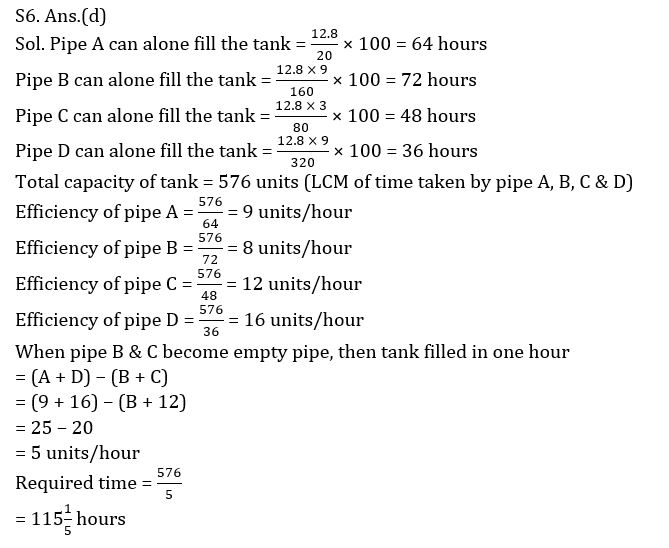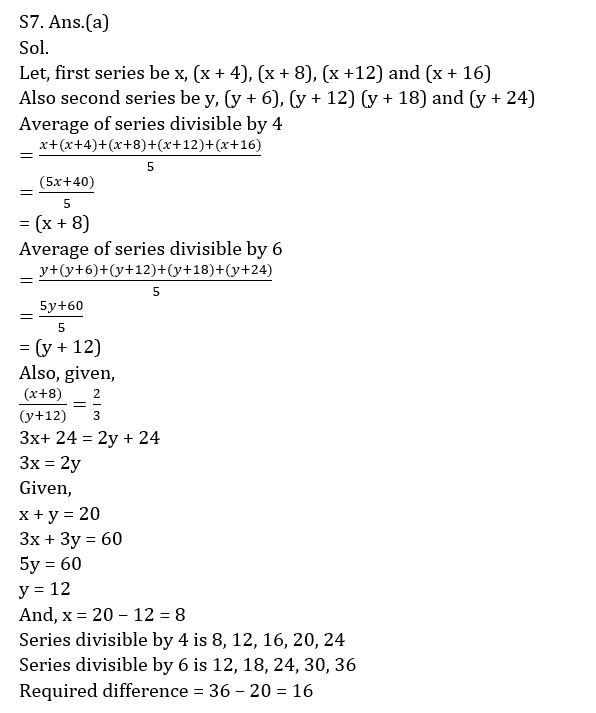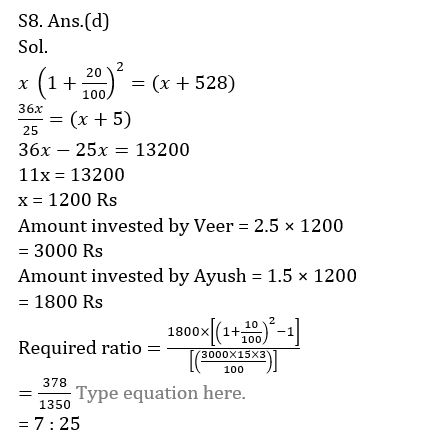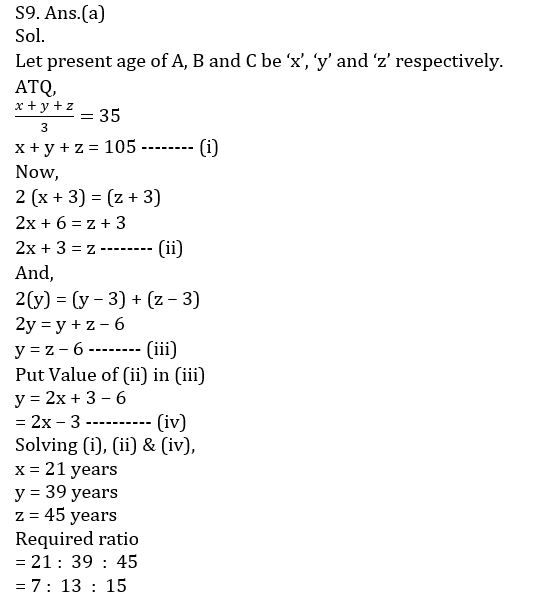## FAQs

### When will the IBPS RRB Clerk Mains Exam be conducted?

IBPS RRB Clerk Mains Exam will be conducted on 16 September 2023.

#### Congratulations!Union Budget 2023-24: Free PDF